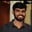Related Tags

python 3
community creator
compound
identity

# What are the compound assignment & identity operators in Python?Buchiredddypalli Koushik

## What are operators in Python?

Operators are special symbols in Python that carry out arithmetic or logical computations. The value that the operator operates on is called the operand. For example: 2+3 = 5. Here, + is the operator that performs addition.

There are many operators in Python, some of them are:

However, in this shot, we will only be talking about the compound assignment operator and the identity operator.

## Compound assignment operator

The compound assignment operator performs the operation of the binary operator on both operands, and stores the result of that operation in the left operand (must be a modifiable value).

• +=
• -=
• *=
• /=
• //=
a = 10
#compound operator
a += 10
print(a)

The above expression is equivalent to a = a+10. In the same way, we can subtract, multiply, and divide a number.

## Identity operator

The identity operator is used to compare objects

Operator Description
is Returns true if both the variables are the same object.
is not Returns true if both the objects are not the same object.
# Example on Identity operator:
x = 10
y = 101
z = x is y
v = x is not y
print(z)
print(v)

z returns False because x is not the same object as y.

v returns True because x is not the same object as y.

RELATED TAGS

python 3
community creator
compound
identity

CONTRIBUTORBuchiredddypalli Koushik
RELATED COURSES

View all Courses

Keep Exploring

Learn in-demand tech skills in half the time